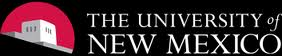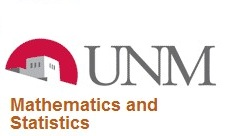## Links to useful statistics websites

Wikipedia                                                                         R
Wolfram MathWorld                                                        SAS
American Statistical Association                                     Matlab
Institute of Mathematical Statistics                                  Maple
The US Census Bureau                                                       SPSS
CDC data and statistics                                                  Minitab
International Statistical Agencies                                    STATA
Statistics Tutorial                                                              S-PLUS

My MS. Thesis:  Bivariate distribution of n iid exponential random variables: KPQ-EXP

My PhD. Dissertation:  On the Equivalence Between the LRT, RLRT and F-test for Testing Variance Components in the Generalized Split-plot Models